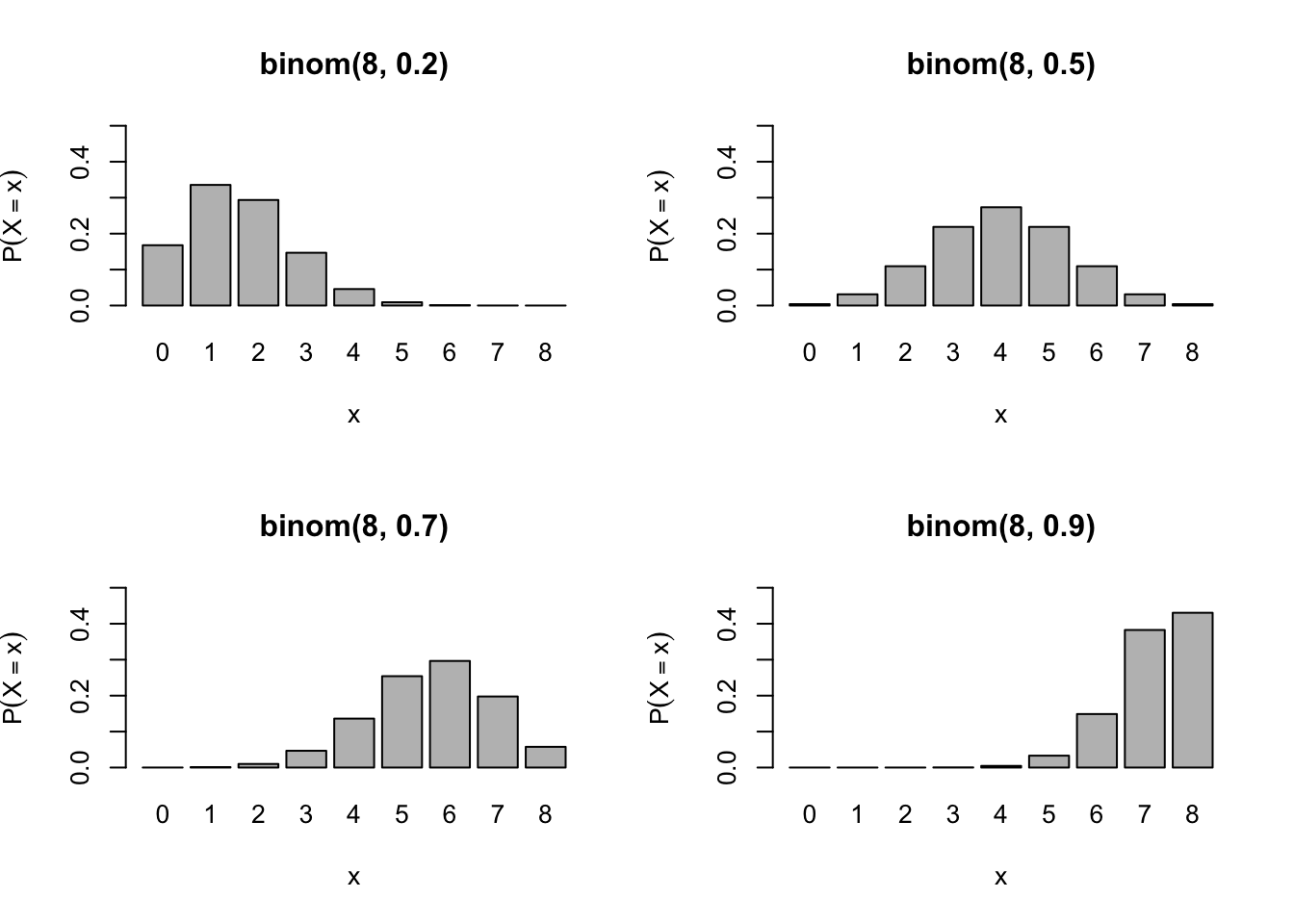These notes are based on Chapter 15 & 16 of Jones, O., Maillardet, R., & Robinson, A. (2009). Introduction to scientific programming and simulation using R. CRC Press.

Building upon probability theory, we are going to study specific probability distributions in these notes. They are so common and/or important that each has got a name and dedicated R built-in functions. Although at some point we may need to create special distributions and write associated functions for our models, it is often sufficient to combine the existing ones as building blocks. So, let’s master them and get prepared for stochastic modelling.

# Discrete distributions

R recognises common distributions by short names and specifies them by parameters.

Distribution R name R Parameters Abbreviation
Bernoulli binom size=1, prob $$\mathrm{Bernoulli}(p)$$
Binomial binom size, prob $$\mathrm{binom}(n,p)$$
Geometric geom prob $$\mathrm{geom}(p)$$
Negative binomial nbinom size, prob $$\mathrm{nbinom}(r,p)$$
Poisson pois lambda $$\mathrm{pois}(\lambda)$$

As you see below, $$\mathrm{Bernoulli}(p)$$ is equivalent to $$\mathrm{binom}(1,p)$$.

R built-in functions for common distributions follow a certain pattern. Suppose that a distribution is recognised by name xyz and specified by parameters p1 and p2, then R provides the following four functions to help you work with the distribution.

• dxyz(x, p1, p2, ...) returns $$P(X=x)$$, the value of PMF at $$x$$.
• pxyz(q, p1, p2, ...) returns $$F(q)$$, the value of CDF at $$q$$.
• qxyz(p, p1, p2, ...) returns $$\operatorname{argmin}_x F(x)\geq p$$, i.e., the quantile function.
• rxyz(n, p1, p2, ...) returns $$n$$ random samples.

In these notes, I avoid using $$p(x)$$ for PMF but use $$P(X=x)$$ to describe the distribution of $$X$$ since we are going to use lots of $$p$$ below.

The support of $$X$$ means the set $$\{x\in\mathbb{R} : P(X=x)>0\}$$ for discrete $$X$$ or $$\{x\in\mathbb{R} : f(x)>0\}$$ for continuous $$X$$ where $$f$$ is a PDF of $$X$$.

## Bernoulli

The support is $$\{0,1\}$$, which is often interpreted as “failure” and “success” respectively. Naturally, it has a single success parameter $$p\in(0,1)$$ that specifies the probability of “success”, i.e. $$p = P(X=1)$$.

Bernoulli distribution serves as a building block and, as you will see below, many complex distributions are modelled as a sequence of independent Bernoulli trials.

\begin{align} P(X=x) &= \begin{cases} p & \text{for } x=1\\ 1 - p & \text{for } x=0 \end{cases}\\\\ \mathbb{E}X &= \sum_{x\in\{0,1\}} xP(X=x)\\ &= 0\cdot(1-p)+1\cdot p\\ &= p\\\\ \operatorname{Var} X &= \sum_{x\in\{0,1\}} (x-p)^2P(X=x)\\ &= (0-p)^2\cdot(1-p) + (1-p)^2\cdot p\\ &= p(1-p) \end{align}

## Binomial

Imagine doing independent $$\mathrm{Bernoulli}(p)$$ trials $$n$$ times. Let $$X$$ denote the number of $$1$$s from $$n$$ trials. Then, $$X\sim \text{binom}(n,p)$$.

Formally, let $$B_1,\dots,B_n \sim \text{Bernoulli}(p)$$. Then, $X = B_1+\dots+B_n .$ Due to the $$n$$ Bernoulli trials, the support is $$\{0,1,\dots,n\}$$.

In deriving the PMF, key computation is to count the number of ways to choose $$x$$ trials that turn out $$1$$ from the total $$n$$ trials, commonly denoted by $$\binom{n}{x}$$.

\begin{align} P(X=x) &= \binom{n}{x}p^x(1-p)^{n-x}\\\\ \mathbb{E}X &= \mathbb{E}(B_1+\dots+B_n)\\ &= \mathbb{E}B_1+\dots+\mathbb{E}B_n\\ &= np\\\\ \operatorname{Var} X &= \operatorname{Var}(B_1+\dots+B_n)\\ &= \operatorname{Var}B_1+\dots+\operatorname{Var}B_n\\ &= np(1-p) \end{align}

$$\operatorname{Var} X$$ formula is drived by exploiting $$n$$ independent Bernoulli trials.

Here are some of PMF plots with varying $$p$$.

n <- 8
x <- 0:n
myplot <- function(p) {
barplot(dbinom(x,n,p),
names.arg = x,
ylim=c(0,.5),
main=paste("binom(",n,", ",p,")", sep=""),
xlab="x",
ylab=expression(P(X==x)))
}
par(mfrow=c(2,2))
for (p in c(.2,.5,.7,.9)) {
myplot(p)
}## Poisson

Imagine doing many independent $$\mathrm{Bernoulli}(p)$$ trials, in each of which success is very rare. For example, the number of car accidents in Melbourne in a single day, where each of thousands of drivers has a very small chance of accident.

While we may use $$\text{binom}(n,p)$$ with large $$n$$ and small $$p$$, we could be in trouble because computers cannot handle extremely large or small values very well. An alternative modelling option is $$\text{pois}(\lambda)$$ with $$\lambda\approx np$$. Here is why.

Let $$Y_n \sim \text{binom}(n,p)$$ such that $$p=\lambda/n$$ for some $$\lambda>0$$. Then,

\begin{align} P(Y_n=x) &= \binom{n}{x}p^x(1-p)^{n-x}\\ &= \frac{n\cdot(n-1)\dotsm(n-x+1)}{x!}\left(\frac{\lambda}{n}\right)^x\left(1-\frac{\lambda}{n}\right)^{n-x}\\ &= 1\cdot\frac{n-1}{n}\dotsm\frac{n-x+1}{n}\frac{\lambda^x}{x!}\left(1-\frac{\lambda}{n}\right)^{n}\left(1-\frac{\lambda}{n}\right)^{-x}\\ &\to 1\cdot 1\dotsm 1\frac{\lambda^x}{x!}e^{-\lambda}1 \text{ as } n\to\infty\\ &= \frac{e^{-\lambda}\lambda^x}{x!} \end{align}

which is the PMF of $$\mathrm{pois}(\lambda)$$. The limit $\lim_{n\to\infty} P(Y_n=x) = \frac{e^{-\lambda}\lambda^x}{x!}$ is called the law of rare events.

As indicated by $$n\to\infty$$, the support is $$\mathbb{Z}_{\geq0}=\{0,1,\dots\}$$.

Both mean and variance are equal to $$\lambda$$.

An important property of $$\text{pois}(\lambda)$$ is the following. Suppose $$X_1,\dots,X_n$$ are independent Poisson random variables with the corresponding parameters $$\lambda_1,\dots\lambda_n$$. Then, $X_1+\dots+X_n = \text{pois}(\lambda_1+\dots+\lambda_n) .$ Here is a proof for $$n=2$$, which lets us generalise to $$n>2$$ by induction.

\begin{align} P(X_1+X_2=n) &= \sum_{m=0}^n P(X_1=m)P(X_2=n-m)\\ &= \sum_{m=0}^n \frac{e^{-\lambda_1} \lambda_1^m}{m!}\cdot\frac{e^{-\lambda_2} \lambda_2^{n-m}}{(n-m)!}\\ &= \frac{e^{-(\lambda_1+\lambda_2)} (\lambda_1+\lambda_2)^n}{n!} \cdot \sum_{m=0}^n\binom{n}{m}\left(\frac{\lambda_1}{\lambda_1+\lambda_2}\right)^m\left(\frac{\lambda_2}{\lambda_1+\lambda_2}\right)^{n-m}\\ &= \frac{e^{-(\lambda_1+\lambda_2)} (\lambda_1+\lambda_2)^n}{n!} \end{align}

because the summand is the PMF for $$\text{binom}\left(n,\frac{\lambda_1}{\lambda_1+\lambda_2}\right)$$.

Let’s compare $$X\sim \text{pois}(\lambda)$$ and $$Y\sim \text{binom}(n,p)$$ such that $$\lambda = np$$. Suppose $$\lambda=1$$ for simplicity and $$n=10^4$$.

lambda <- 1
n <- 1e4
x <- c(0,2,5)
$$x$$ $$P(X=x)$$ $$P(Y=x)$$
0 0.3678794 0.367861
2 0.1839397 0.1839489
5 0.0030657 0.003064

Even with a moderate $$n$$, Poisson provides reasonable approximation of Binomial.

Here are some of PMF plots with varying $$\lambda$$.

n <- 12
x <- 0:n
myplot <- function(l) {
barplot(dpois(x,l),
names.arg = x,
ylim=c(0,.6),
main=paste("pois(",l,")", sep=""),
xlab="x",
ylab=expression(P(X==x)))
}
par(mfrow=c(2,2))
for (l in c(.5,1,2,5)) {
myplot(l)
}